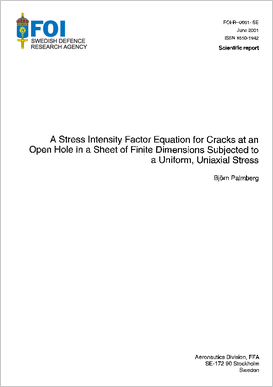# A stress intensity factor equation for cracks at an open hole in a sheet of finite dimensions subjected to a uniform, uniaxial stressAuthors:

Publish date: 2001-01-01

Report number: FOI-R--0061--SE

Pages: 80

Written in: English

## Abstract

In many applications it is more convenient to have an equation for computing the stress intensity factor instead of interpolating in tables or reading values from graphs. This is particularly true if there are many parameters involved as in the case of radial cracks at a circular hole in a rectangular sheet. The aim of this investigation is to develop a stress intensity factor equation for cracks at a hole in a limited sheet. It is assumed that the sheet is loaded along the edges parallel to the cracks with a uniform, unaxial stress. The aim is also to assess the accuracy of the equation through comparisons to different numerical solutions. An equation consisting of five parts has been developed. The first part is the stress intensity factor for cracks at a hole in an infinite sheet. The second part is a finite width correction. The third part makes a correction for eccentricity. The fourth part represents a finite height correction. Finally, the fifth part includes a correction for interaction between the hole and the finite height. The accuracy of the stress intensity factor equation is generally better than 20% and in most cases better than 8%.

Share page on social media